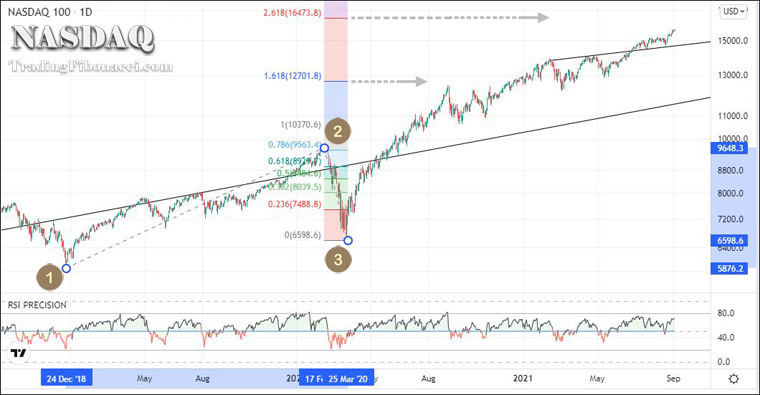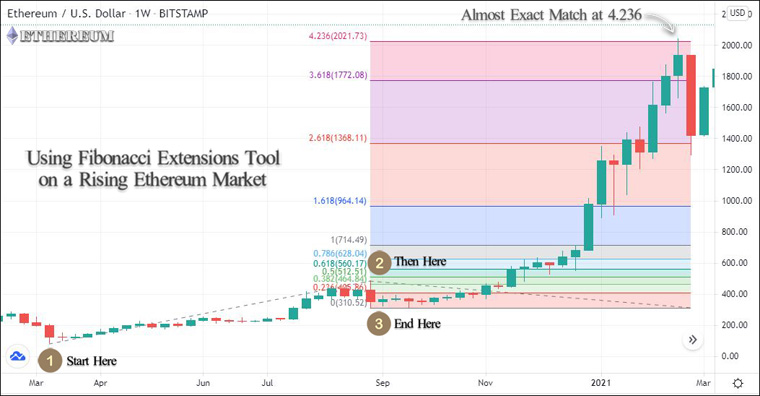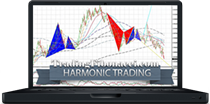How to Use the Fibonacci Extensions Tool

The Fibonacci Extensions is a multi-timeframe trading tool that can be applied to any financial market. What makes it unique is that it can show exact take-profit levels when an asset breaks an all-time high (ATH) and enters the price-discovery territory.

What can the Fibonacci Extensions show?

While the Fibonacci Retracement shows potential levels of price retracement, Fibonacci Extensions show how high the price can go following a retracement. Fibonacci Extensions are quite useful for spotting market tops when an asset is in a price-discovery territory. However, the tool can also be used for spotting market bottoms.

Fibonacci Extensions calculate the impulse wave in the same direction as the trend. You can customize the tool to show any levels, however, you can use the levels presented below.

Table: Key Fibonacci levels

 Key Fibonacci Levels 0.2360 1.0000 0.3820 1.6180 0.5000 2.6180 0.6180 3.6180 0.7860 4.2360

How to use the Fibonacci Extensions tool

The Fibonacci Extensions tool consists of a basic trendline, the extension line, and the Fibonacci retracements. In order to draw effectively the ‘Fibonacci Extensions’, you need three points:

(i) Start of the impulse wave (trendline)

(ii) End of the impulse wave (trendline), the start of the retracement (extension line)

(iii) End of the retracement (extension line)

(i) Start of the impulse wave

• The price corresponds to the starting point of the trendline.
• This point shows the beginning of a new price trend.
• It can be a swing high (top) or a swing low (bottom).
• After this point, there is a strong price trend.

(ii) End of the impulse wave

• The price corresponds to the endpoint of the trendline.
• This point shows the beginning of the reversal.
• After this point, there is a price retracement.

(iii) End of the retracement

• The price corresponds to the end of the extension line.
• This point shows the end of the price retracement.
• After this point, the price reverses in the original direction.

After selecting the tool, click the first point, at the start of the impulse wave (Point-1), the second point, at the end of the impulse wave (Point-2), and the third point, at the end of the retracement (Point-3).

Examples when trading with Fibonacci Extensions -Taking profit

One of the most challenging tasks in financial trading is determining where to take profits. Things get even more complicated when the price breaks an all-time high (ATH) and enters the price-discovery territory. In this case, Fibonacci Extensions can pinpoint target levels where the price is likely to reverse.

• Alternatively, you can ride the trend using a trailing stop.

Using the Fibonacci Extensions on Nasdaq (US100)

In the following chart, the tool is applied to Nasdaq during the bull market of 2019-2021.

Chart: Applying Fibonacci Extensions to the daily Nasdaq (US100)Using the Fibonacci Extensions on Ethereum

In the following chart, the tool is applied to ETHUSD during the 2020-2021 bull run. After creating a new all-time high, the price of Ethereum was in a price-discovery territory.

Chart: The Fibonacci Extensions tool and EthereumAs shown in the above ETHUSD chart, the target of 4.236 was almost an exact match.

Notes:

• When the price of an asset enters the price-discovery territory, the most important extensions levels are 1.618, 2.618, 3.618, and 4.2360.
• The Ethereum chart is found in the book “Bitcoin & Ethereum Trader” by George Protonotarios. ► Find the book on Amazon: https://www.amazon.com/dp/B09DQ54897

■ Fibonacci Extensions Tool

Online Forex BrokersFibonacci mathematics aims to reveal the hidden proportionality of market behavior. Find Fibonacci trading tools and tutorials:

eBOOKTRADING WORLD MARKETS USING PHI AND THE FIBONACCI NUMBERS (2018)

Complete Guide to Fibonacci Trading with Reference to Elliott Waves, Gann Numbers, and Harmonic PatternsForex Rebates

Start trading Forex and receive rebates for every trade you execute. Permanent participation, 100% free of any charge. Contact Forex Rebates today..Chart PatternsIdentify and Trade Reliable Chart Patterns: